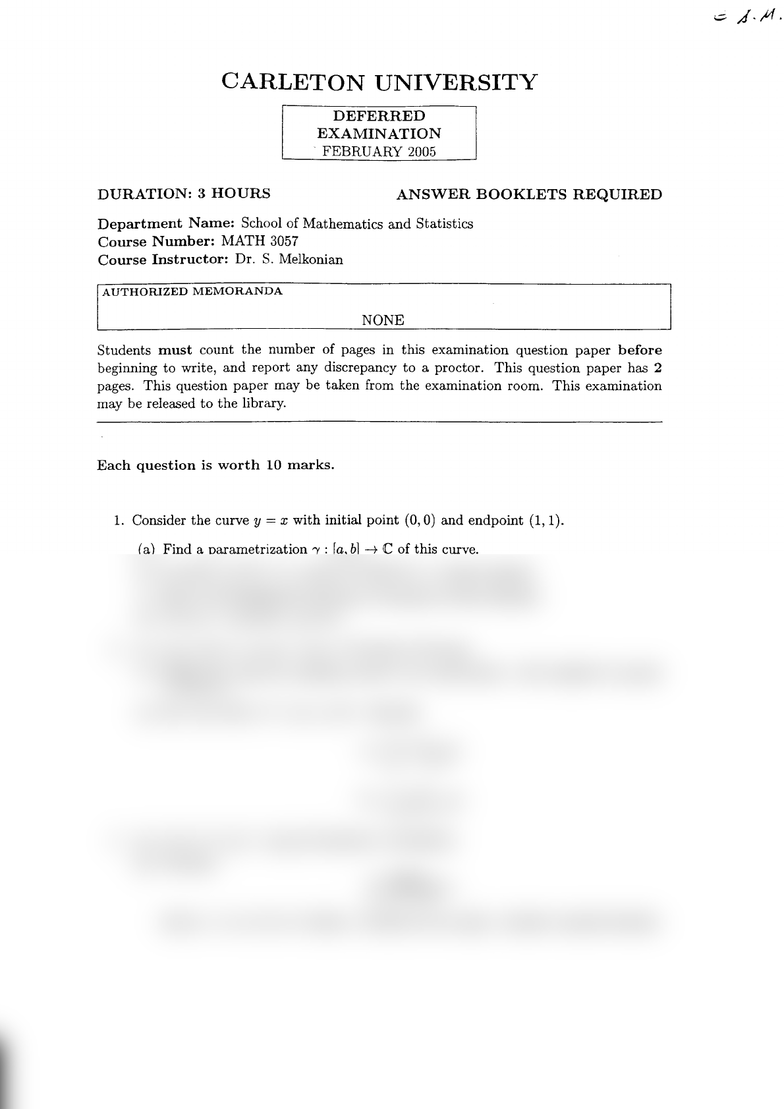Study Guides (390,000)
CA (150,000)
Carleton (5,000)
MATH (200)
All (1)
Midterm

# Exam-MATH3057-2005FebruaryExam

Department
Mathematics
Course Code
MATH 3057
Professor
All
Study Guide
Midterm

This preview shows half of the first page. to view the full 2 pages of the document.CARLETON UNIVERSITY
DEFERRED
EXAMINATION
FEBRUARY
2OO5
DURATION: 3 HOURS ANSWER BOOKLETS REQUIRED
Department Name: School
of
Mathematics
and
Statistics
Course Number: MATH 3057
Course fnstructor: Dr. S. Melkonian
AUTHOzuZED MEMORANDA
NONE
Students must count the number of pages in this examination question paper before
beginning
to write, and report any discrepancy
to a proctor. This question paper has 2
pages. This question
paper may be taken from the examination room. This examination
may be released to the library.
Each question is worth 10 marks.
1. Consider
the curve A
: r with initiai point (0,0) and endpoint (1,
1).
(a) Find a parametrization "y
: [a,b]
-+ C of this curve.
(b) Evaluate [1322 dz by using the definition of a line integral.
(c) State the fundamental theorem of Calcuius for line integrals.
(d) Use
(c)
to evaluate
[^,322
dz.
2. (a) State the homotopy version
of Cauchy's
theorem.
(b) Define the inder (or wind,ing
number) of a closed
curve
7 with respect to a point
z0 not on'y.
(c) Let lQ) :2i + ett, 0 < , < 2n. Evaluate
fr
(rl | _ "rdz
J1 a - aL
f1
(ii)
I zzandz
3. (a) State Cauchy's
integral formula for derivatives.
(b) Evaluate
/ sin(z)
1,6
-
4o''
where
7 is the circle of radius 4 centred
at the origin, oriented counterclockwise.
= ,!'/{ '